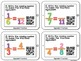# FractionsSubject
Resource Type
File Type

PDF

(9 MB|28 pages)
Standards
• Product Description
• StandardsNEW
Fractions can be fun with these 5 engaging sets of fraction Scan the Room activities. Students must roam around the room solving fractions problems. Students get immediate feedback when they scan the QR code. A student recording sheet and answer keys are included. ALL 3rd grade CCSS fractions standards are covered as well as several 4th grade standards. Listed below are the fractions skills included and their Common Core alignment.

-Identifying Fractions 3.NF.1
-Fractions on a Number Line 3.NF.2a, 3.NF.2b, 3.NF.3c
-Equivalent Fractions 3.NF.3a, 3.NF.3b, 4.NF.1
-Comparing Fractions 3.NF.3d, 4.NF.2
-Adding and Subtracting Fractions 4.NF.3a, 4.NF.3d

Please download the preview to get a closer look at the sets. Each set includes 16 cards. Please make sure you have a device that can scan QR codes before purchasing.

*I have this same exact product without QR codes HERE

Be sure to check out my Scan The Room: Numbers and Operations in Base 10 Set
Solve word problems involving addition and subtraction of fractions referring to the same whole and having like denominators, e.g., by using visual fraction models and equations to represent the problem.
Understand addition and subtraction of fractions as joining and separating parts referring to the same whole.
Compare two fractions with different numerators and different denominators, e.g., by creating common denominators or numerators, or by comparing to a benchmark fraction such as 1/2. Recognize that comparisons are valid only when the two fractions refer to the same whole. Record the results of comparisons with symbols >, =, or <, and justify the conclusions, e.g., by using a visual fraction model.
Explain why a fraction 𝘢/𝘣 is equivalent to a fraction (𝘯 × 𝘢)/(𝘯 × 𝘣) by using visual fraction models, with attention to how the number and size of the parts differ even though the two fractions themselves are the same size. Use this principle to recognize and generate equivalent fractions.
Compare two fractions with the same numerator or the same denominator by reasoning about their size. Recognize that comparisons are valid only when the two fractions refer to the same whole. Record the results of comparisons with the symbols >, =, or <, and justify the conclusions, e.g., by using a visual fraction model.
Total Pages
28 pages
Included
Teaching Duration
N/A
Report this Resource to TpT
Reported resources will be reviewed by our team. Report this resource to let us know if this resource violates TpT’s content guidelines.